UP

# Comparison of Evolutionary Algorithms on a Benchmark Function Set

The Task. Black-box optimization of 25 benchmark functions under thoroughly defined experimental and recording conditions for the 2005 IEEE Congress on Evolutionary Computation: Session on Real-Parameter Optimization (see resources links below). 17 papers were submitted, 11 were accepted, thereunder hybrid methods.

Algorithms. We submitted two algorithms.

• L-CMA-ES (Auger and Hansen 2005b): A CMA evolution strategy with small population size and small initial step-size to emphasize on local search characteristics. Independent restarts are conducted until the target function value is reached or the maximum number of function evaluations is exceeded.
• G-CMA-ES (Auger and Hansen 2005a): A CMA evolution strategy restarted with increasing population size (IPOP). Independent restarts are conducted with increasing population size until the target function value is reached or the maximum number of function evaluations is exceeded. With the initial small population size the algorithm converges fast, with the succeeding larger population sizes the global search performance is emphasized in subsequent restarts.

Results. The following figures show the comparison of performance results from the 11 algorithms for search space dimension 10 and 30 on different function subsets. The expected number of function evaluations to reach the target function value is denoted with FEs (equivalent to the performance measure SP1 in Auger and Hansen 2005b) and normalized by the value of the best algorithm on the respective function, FEsbest. Shown is, for each algorithm, the empirical cumulative distribution function of FEs / FEsbest over different sets of functions in 10 and 30D. Small values for FEs / FEsbest and therefore large values of the graphs (the cumulative distribution function) are preferable.
All functions solved by at least one algorithm Unimodal functions Multimodal functions
10D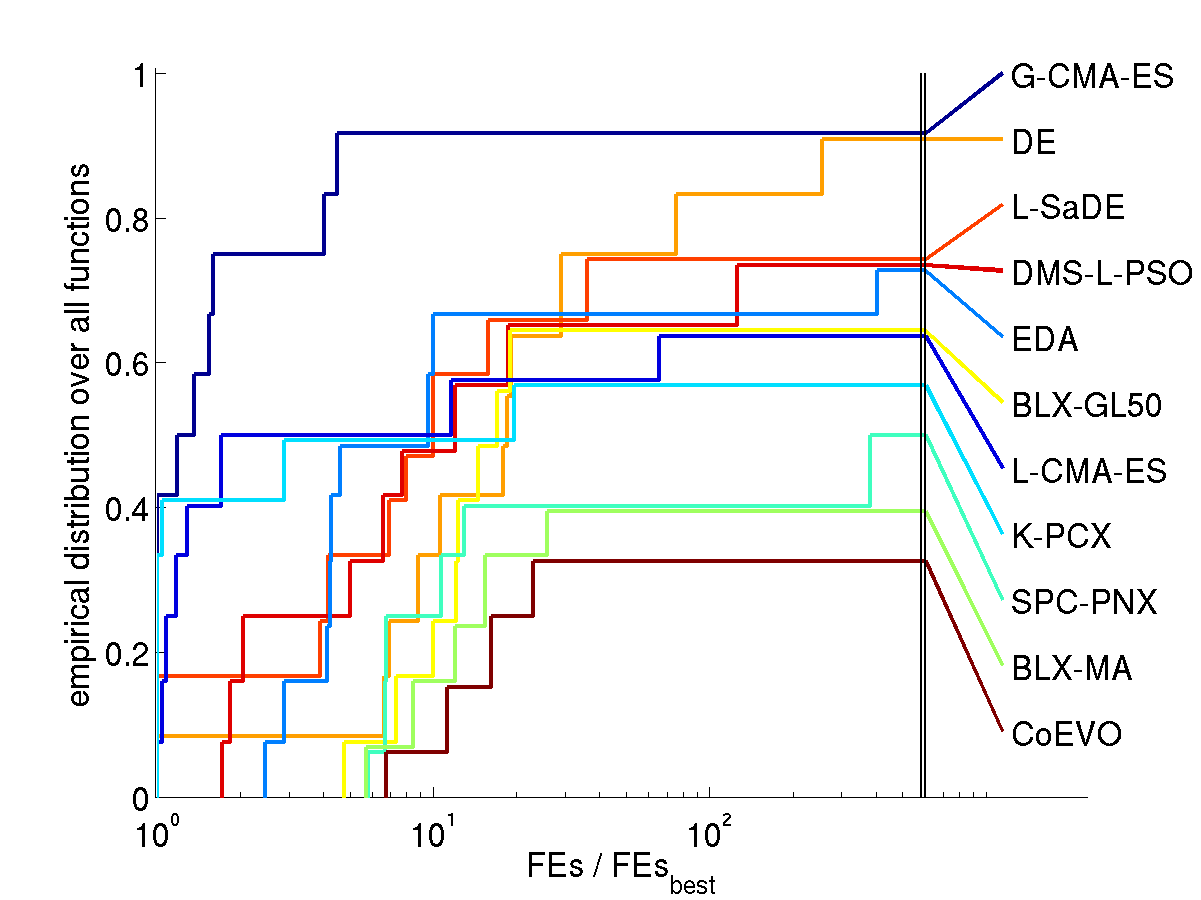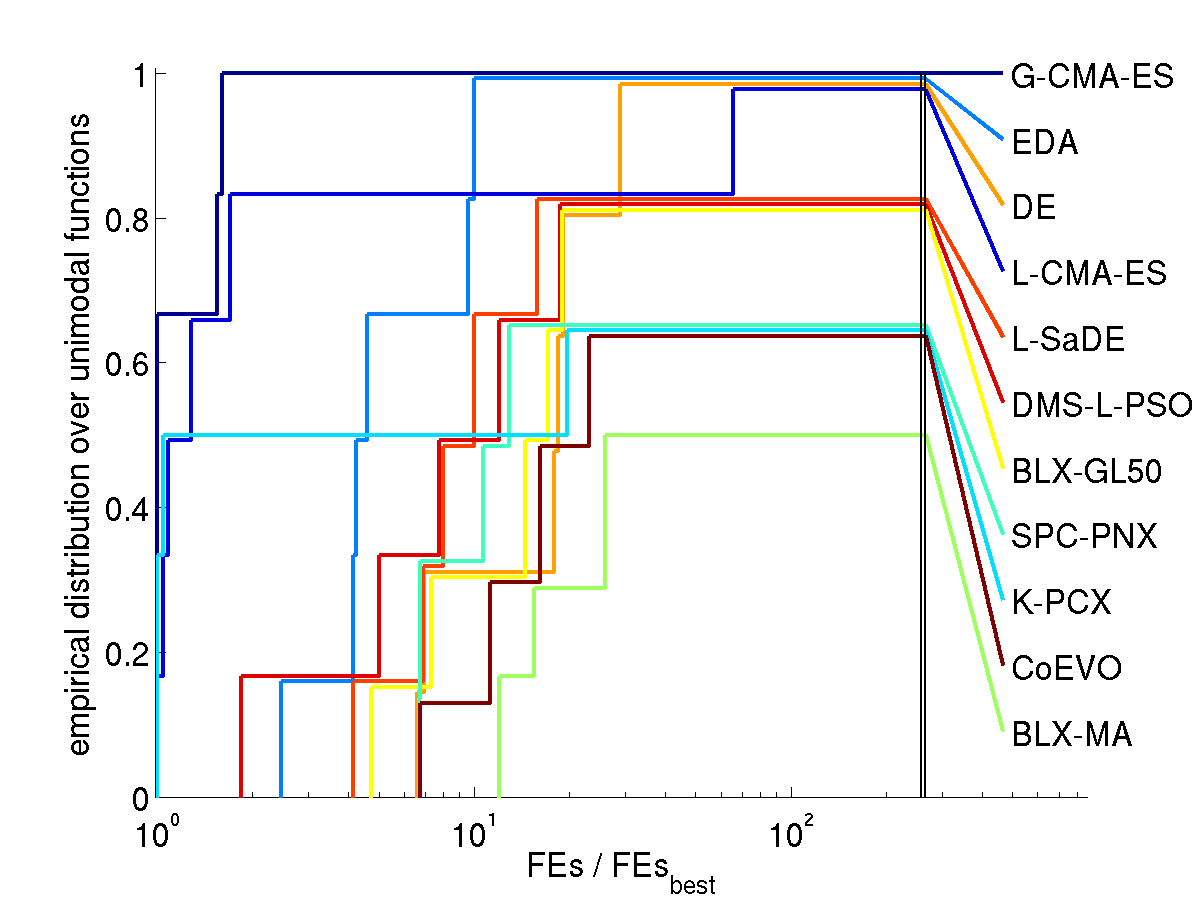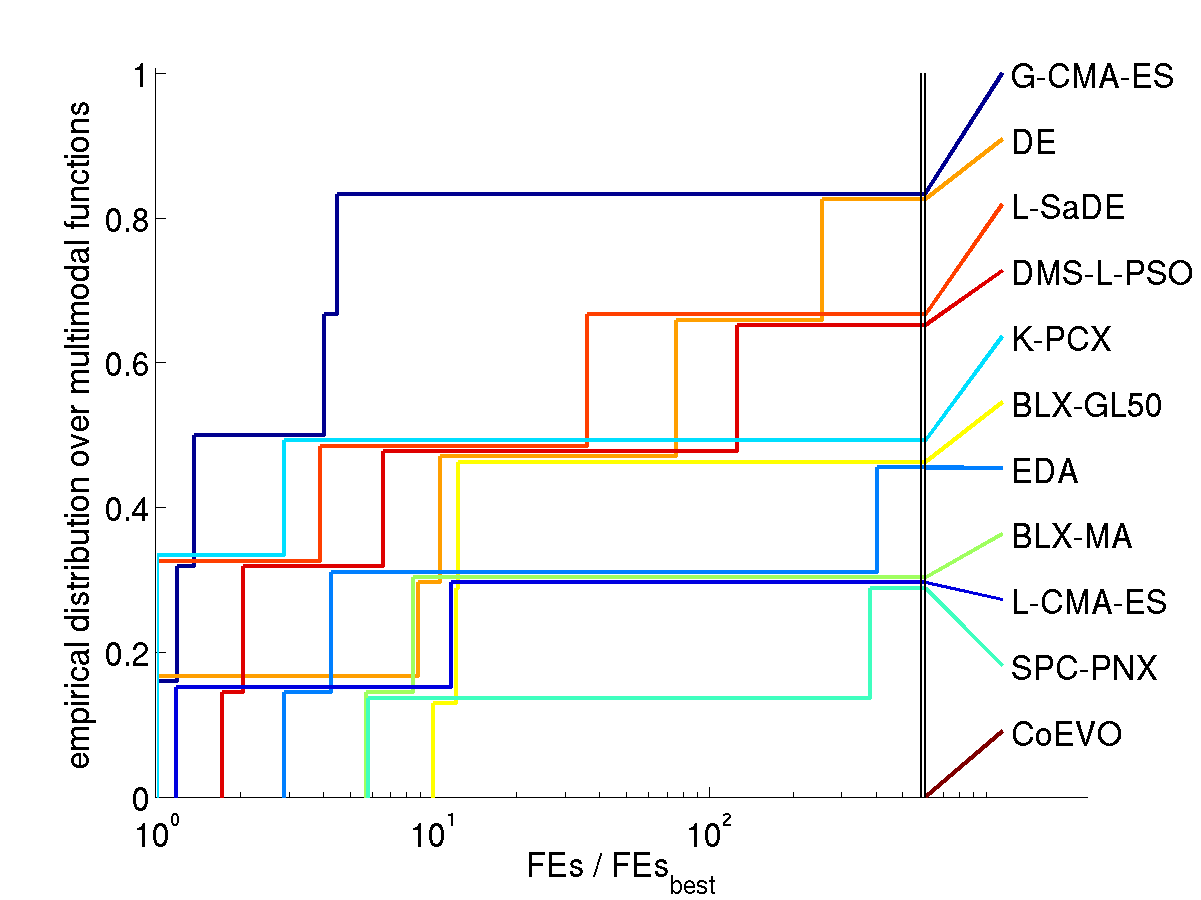30D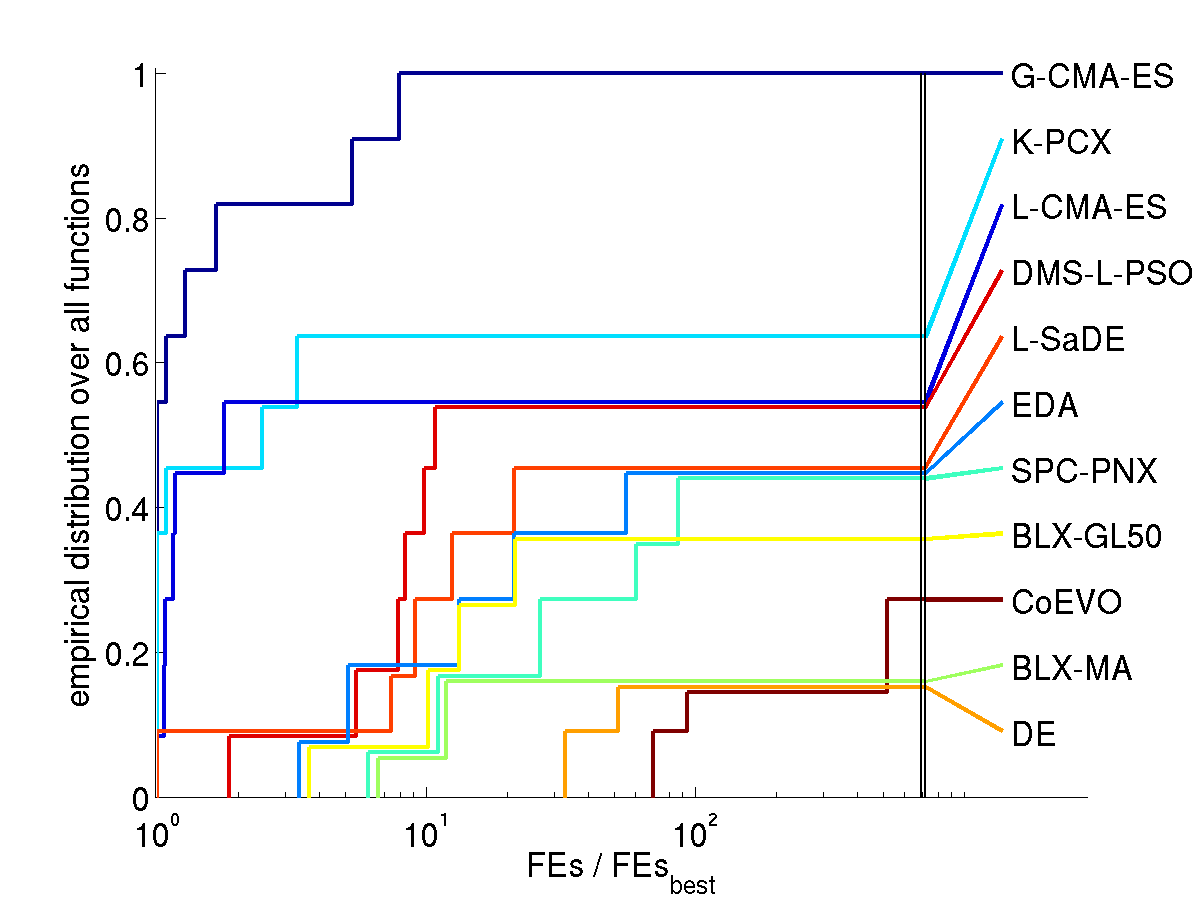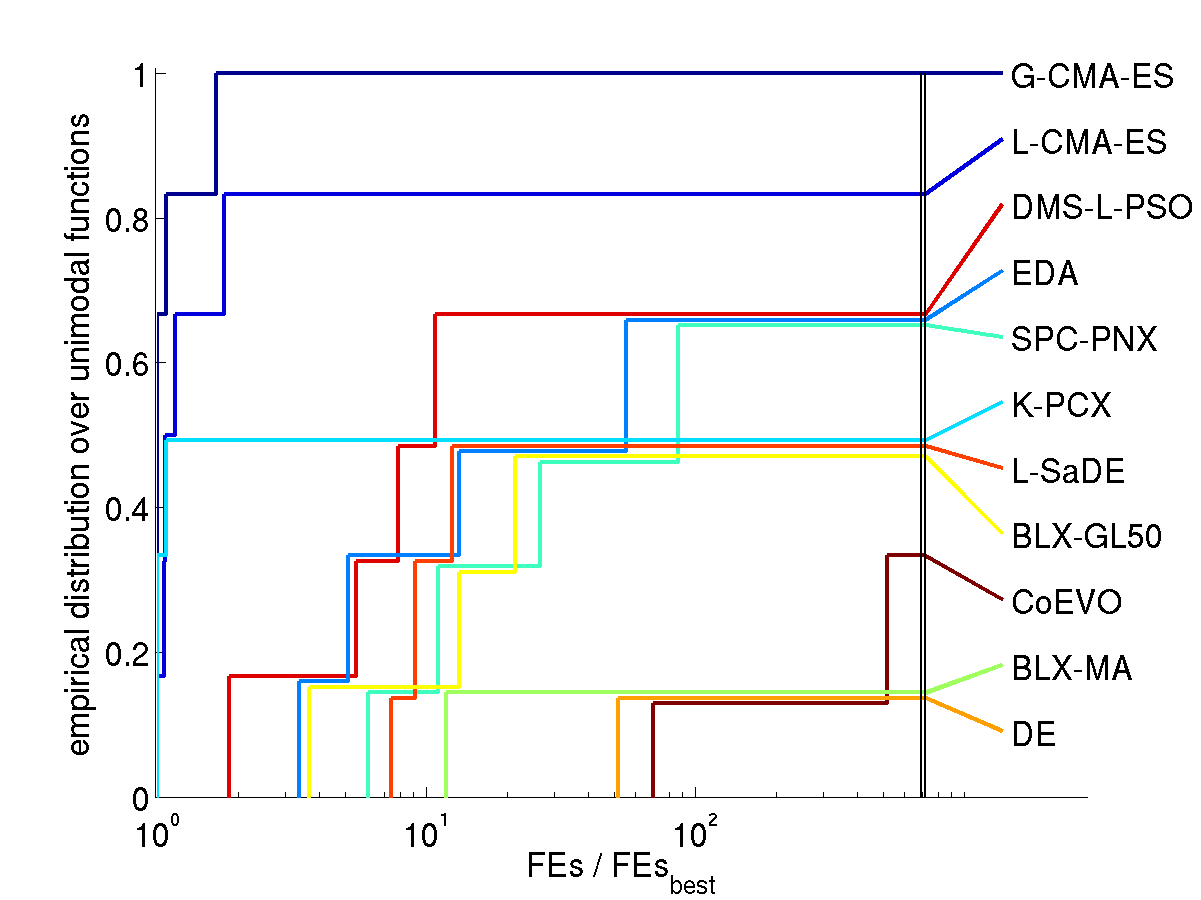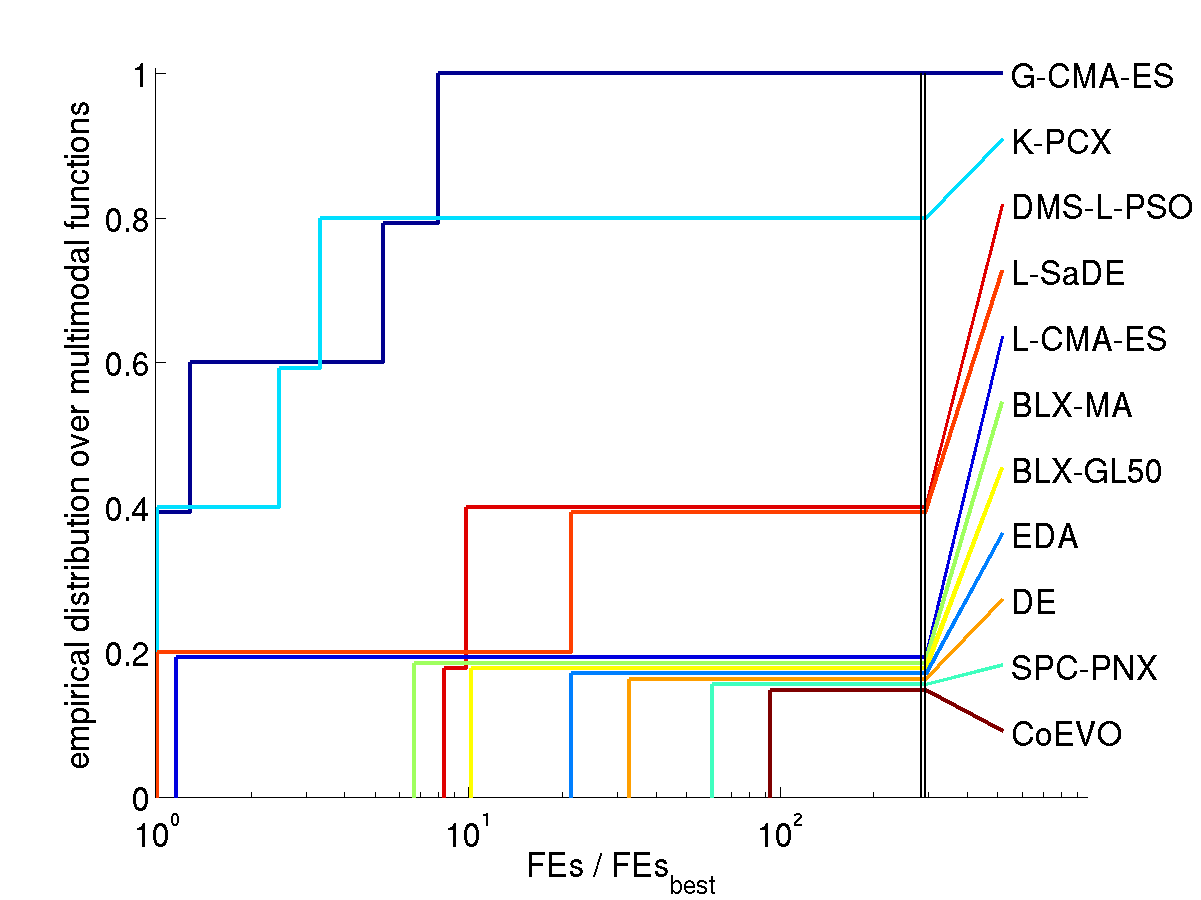The algorithms are referenced in the comparison of results in the Resources section below.

How to read the graphs. In the lower left figure (all solved functions in 30D) we find for FEs / FEsbest = 2 (x-axis) a value of about 0.82 (y-axis) for the graph of G-CMA-ES (uppermost graph). That means, on 82% of the functions G-CMA-ES was at most 2 times slower than the best algorithm (in terms of expected number of function evaluations). For FEs / FEsbest = 10 we find a value of 1 in the distribution graph, meaning that G-CMA-ES was maximum 10 times slower on all of the functions. The right most values of the graphs give the ratio of ever solved functions, ranging here between 100% for G-CMA-ES and just under 20% for BLX-MA and DE (due to the comparatively low number of overall allowed function evaluations).

Discussion. Compared to all contributed algorithms the G-CMA-ES appears to be advantageous from three perspectives.

1. The G-CMA-ES performes best in terms of number of function evaluations to reach the target function value
• over all solved functions (left figures)
• on the subset of unimodal functions (middle figures)
• on the subset of solved multimodal functions (right figures)
The G-CMA-ES performes best, in terms of ranking of the final function value, on the remaining subset of functions, where no algorithm reached the target function value in any trial (see comparison of results in the Resources section below). On two separable functions the CMA-ES is considerably outperformed by other contributed algorithms.
2. No parameter tuning is conducted for the CMA-ES which appears to be true also for L-SaDE, BLX-MA, DMS-L-PSO, BLX-GL50, and CoEVO. The default parameter values were applied for CMA-ES.
3. The CMA-ES exhibits the most invariance properties together with EDA.

Interpretation. Hypothetical explanations for the performance advantage of the CMA-ES are the following (still valid in June 2011).

1. On unimodal functions:
• The population size is small in the beginning.
• The population can get an (arbitrarily) elongated shape along any direction in search space without collapsing into a lower dimensional subspace even for small population size.
• Step-size control effectively prevents premature convergence and allows for fast convergence to an optimum. This is not a contradiction in terms.
2. On multi-modal functions the population spread is decisive.
• Even for a small population size the population spread is comparatively large, due to the cumulative step-size adaptation (path length control), and hinders convergence to the very closest local optimum.
• Larger population sizes are used after each restart. The larger population sizes can facilitate a larger population spread for longer time periods and therefore a "more global" search. Here, the covariance matrix update facilitates a comparatively large population spread.

Remarks.

• The CMA-ES was the only non-elitistic algorithm in the competition.
• There has been an inconsistency with the boundary handling in the experimental setup, which is, at least in part, now documented in the respective batch files. On some functions, boundary handling has a significant impact on the performance.

References

1. Auger, A, and Hansen, N. (2005a). A Restart CMA Evolution Strategy With Increasing Population Size. In Proceedings of the IEEE Congress on Evolutionary Computation, CEC 2005, pp. 1769-1776 (abstract & erratum, paper in pdf).
2. Auger, A, and Hansen, N. (2005b). Performance Evaluation of an Advanced Local Search Evolutionary Algorithm. In Proceedings of the IEEE Congress on Evolutionary Computation, CEC 2005, pp.1777-1784 (abstract & erratum, paper in pdf).

Acknowledgment We are extremely grateful to Stefan Kern for his contribution to this work.

UP
updated June, 2011